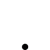1 svar
45 visningar

solow residual

Hej! Jag har denna uppgiften att göra:

The aim of this paper is to compare the growth experiences of two different countries. Select two countries for which you think a growth comparison would be interesting.

Restrict your choice to countries for which the capital stock series are available and have at least 30 years of data.

You need to choose which measure of GDP, Employment and Capital you should use.
This is mainly an over time within country comparison. You should not compare levels of GDP or productivity and don’t need work with PPP adjusted numbers.

Identify the contributions of capital accumulation and productivity growth to growth. Construct
the Solow residuals using the following equation:

Growth in Y/L = alpha*( Growth in K/L ) + Solow Residual

calculated as

Ln(Y(t)) -Ln(L(t))  -  (  Ln(Y(t-1)) - Ln(L(t-1))  )  =  growth in Y/L

alpha*  ( Ln(K(t)) -Ln(L(t))   -  (  Ln(K(t-1)) - Ln(L(t-1)) )  =  alpha*growth in K/L

The difference between the above numbers= solow residual

Assume alpha = 0.33, which is a common estimate for the share of capital.

Make two graphs. The first should compare the growth rates in Y/L of the two countries. The second
should plot the three components of the equation above to see how growth is broken down into
two components: the part that comes from capital accumulation (building more machines) and
the part that comes from productivity improvements (other than from capital accumulation).

Mitt problem är att jag inte vet vilka siffror jag ska använda mig av i formeln för att kunna räkna ut rätt svar.

Tack på förhand:-)

Vilka länder har du valt? Det kan vara smart att försöka leta upp statistiken först och välja två av de länder som du hittar bäst statistik för.

Avbryt
Close